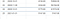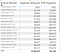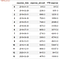# Three Routes: YTD and Cumulative Sum

## Relevant Scenario(s)

When you are accumulating wealth, you will run into the task of aggregating and analyzing the totals, either per period or over an entire span of time.

General Ledger Account Scenario

In the case temporary accounts such as expenses, which is a temporary general ledger account, an accounting firm will look to re-calculating the expenses every year, since its account balance is removed by transferring the amount to another account. The value resets with the start of the next fiscal year and accumulates once again until the end of that fiscal year.

Retail Sales Amount scenario

Then there are scenarios where you want the aggregation to span over years. For instance, if you might want to aggregate your sales amount over all the years since you established your retail store.

In this article we look into the differences in the techniques that cover both scenarios — YTD amounts that reset with the start of the next year and aggregations that span over periods.

Three techniques have been provided below which create the output desired using a relational database, Pandas and Power BI- with usage of DAX functions.

## Data table

The data we will consider here is an excel sheet with two columns as seen in Fig 1. The data is a running list of dates and amounts. The amounts will represent the expense amount of our General Ledger amount scenario as well as the sales amount of our Retail Sales scenario. With the same set of values, we will see the difference in generating a running total across multiple years and a total that resets every fiscal year end and aggregates beginning from 0 at the start of the next fiscal year. We will assume the fiscal year in this case to coincide with the Gregorian calendar.

Fig 1:

## Technique in SQL

PostgreSQL
We will create two database tables to hold the data for the two scenarios described above. “retail_sales” will contain the sales data and “gla_expense” table will contain the expense data. We will then load the data directly from the excel file into the database using a script of inserts created from the data in the excel file. See section Setup Data section at the very bottom of this article for details. We choose this approach of generating an Insert statements script since we have only a few rows of data to load. CSVs can also be loaded using the Import/Export feature in pgAdmin. You can find more details about loading data from a CSV file in the Setup Data section of this article.

1. Running total over multiple years without a reset

When accumulating the total sales over years, we aggregate using a windowing function without any partitioning. The summation over an ascending “order by” of the sales_date, effectively sums up the sales_amount in a cumulative fashion as it goes through the dates even when the year switches from 2019 to 2020.

Similarly when the date transitions into the year 2021, the amount continues to aggregate:

2. Expense Amount year to date aggregation with year end reset
With a little tweak of the query above we can break the accumulation of expense so that the value starts aggregating from 0 at the beginning of the every fiscal year. Partitioning the aggregation window by years while summing the expense by ascending expense date produces the desired result.

Notice that in the above screen shot, the aggregation stops at 2019 and resumes with the total for January 2019 equal to the expenses in that month alone.

And similarly when the year transitions to 2021, we see the amount starts aggregating over again with the amount in January 2021 as the first month end total.NOTE THE TRANSITION FROM DECEMBER 31, 2020 to JANUARY 31 2021

## Technique in Power BI

Setting up the data in Power BI is accomplished rather easily by loading the CSV using Get Data->Text/CSV. The queries are renamed as retail_sales and gla_expense in keeping with the database tables.

In the Modeling tab, connect the dimension date table that spans the entire time period of the expense and sales dates with a row for each date in that span.

1. Running total over multiple years without a reset

As seen in the matrix visualization below, for the sales amount we want a continuous aggregation spanning multiple years. After creating a measure for the total sales:

The measure is used in measure for the Cumulative sum called Running Total

The DAX starts with declaring a variable for the visible MAX date from the DimDate table which is marked as the Date table. As mentioned above this query has all dates for the years that expenses and sales span, and not just the dates in the retail_sales or gla_expense tables.

Once the MAX date has been captured (this is the current row in the matrix), the CALCULATE function is used to evaluate an expression by adding the filtered set attained by :
a) grabbing all the dates from the dimension table and then
b) filtering down to those dates that are less than or equal to the current date in the row.

This results in the sales amount being summed up for each row as the sum of all amounts prior to the date in the row. The running total is just accumulates values as it runs down the dates all the way up to the last date in the retail_sales query.

2. Expense Amount year to date aggregation with year end reset

The data in the CSV file is stored in the query gla_expense

Now the matrix visualization can be created using the Date from the DimDate table, the Expense Amount and the DAX measure:

In contrast to the sales running total, you will notice that with the start of a fiscal year the YTD expense below, starts with the total for the first month of the fiscal year.

This is accomplished by using the DATESYTD DAX function shown above applied on a tables of all dates in the dimension date table less than the maximum limit of dates in the expense table. The measure YTD expense now aggregates the expenses over the dates in a year and then start over with the new fiscal year.

TIP: The DATESYTD also accepts a month end parameter that makes specifying your fiscal year end date very convenient.NOTE THE TRANSITION in YTD Expense FROM DECEMBER 31, 2019 to JANUARY 31 2020

Notice how the YTD Expense resets at the beginning of each fiscal year — 2020 and 2021?

## Technique in Pandas

Data in the CSV file is imported into Jupyter Notebook using Pandas read_csv . Before manipulating the data to get he running totals, we modify the types of the date and amount columns and appropriately set them to date and numeric respectively.

1. Running total over multiple years without a reset

Ensure that the data read in has the right type. The date is a Pandas datetime. The sales amount is numeric.

The above code uses cumsum function that aggregates the sales_amount. Our data is sorted in ascending order of sales_date. If the data is not sorted use sort_values(by=’sales_date’) function before applying cumsum function. The result visible below is continuous aggregation all the way to the end.

2. Expense Amount year to date aggregation with year end reset

Ensure that the data read in has the right type. The date is a Pandas datetime. The sales amount is numeric.

To accomplish the partitioning by year so that aggregation resets with each fiscal year, we first group by the date field by year. Then the cumsum function operates on amounts in a each year group.

And so we get the aggregation of 2019, followed by a new set of aggregations for 2020.NOTE THE TRANSITION in YTD expense FROM DECEMBER 31, 2019 to JANUARY 31 2020

# Setup data

CSV files can be imported into PostgresSQL (pgAdmin) , Pandas and Power BI. For the purpose of this demo, data was created in Excel using Excel’s RANDBETWEEN function.

The same data can be loaded into the tables for expense amount and retail sales amount.

Example Script in PostgreSQL

To load the data into the database from an Excel file.

1. Create a tables with the column names for the data to be imported in the Postgres database.

Copy the INSERT statements into the Query editor in pgAdmin and run the Inserts.

To capture the data into a Jupyter notebook: